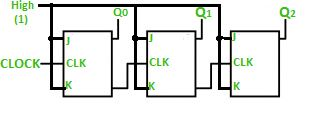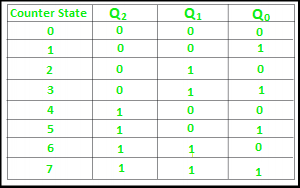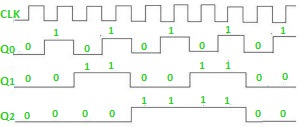# Ripple Counter in Digital Logic

Counters play a crucial role in digital logic circuits, enabling tasks such as clock frequency division and sequencing. This article explores the concept of ripple counters, a type of asynchronous counter, their operation, advantages, and disadvantages in digital logic design.

## What is a Counter?

Counter is basically used to count the number of clock pulses applied to a flip-flop. It can also be used for Frequency divider, time measurement, frequency measurement, distance measurement, and also for generating square waveforms. In this, the flip-flops are asynchronous counters and are supplied with different clock signals, there may be a delay in producing output. Also, a few numbers of logic gates are needed to design asynchronous counters. So they are elementary in design and also are less expensive.

## What is a Ripple counter?

Ripple counter is a cascaded arrangement of flip-flops where the output of one flip-flop drives the clock input of the following flip-flop. The number of flip flops in the cascaded arrangement depends upon the number of different logic states that it goes through before it repeats the sequence a  parameter known as the modulus of the counter. A n-bit ripple counter can count up to 2n states. It is also known as MOD n counter. It is known as ripple counter because of the way the clock pulse ripples its way through the flip-flops. Some of the features of ripple counter are:

• It is an asynchronous counter.
• Different flip-flops are used with a different clock pulse.
• All the flip-flops are used in toggle mode.
• Only one flip-flop is applied with an external clock pulse and another flip-flop clock is obtained from the output of the previous flip-flop.
• The flip-flop applied with an external clock pulse act as LSB (Least Significant Bit) in the counting sequence.

A counter may be an up counter that counts upwards or can be a down counter that counts downwards or can do both i.e.count up as well as count downwards depending on the input control. The sequence of counting usually gets repeated after a limit. When counting up, for the n-bit counter the count sequence goes from 000, 001, 010, … 110, 111, 000, 001, … etc. When counting down the count sequence goes in the opposite manner: 111, 110, … 010, 001, 000, 111, 110, … etc.

A 3-bit Ripple counter using a JK flip-flop is as follows:In the circuit shown in the above figure, Q0(LSB) will toggle for every clock pulse because JK flip-flop works in toggle mode when both J and K are applied 1, 1, or high input. The following counter will toggle when the previous one changes from 1 to 0.

Truth Table is as follows:The 3-bit ripple counter used in the circuit above has eight different states, each one of which represents a count value. Similarly, a counter having n flip-flops can have a maximum of 2 to the power n states. The number of states that a counter owns is known as its mod (modulo) number. Hence a 3-bit counter is a mod-8 counter. A mod-n counter may also be described as a divide-by-n counter. This is because the most significant flip-flop (the furthest flip-flop from the original clock pulse) produces one pulse for every n pulses at the clock input of the least significant flip-flop (the one triggers by the clock pulse). Thus, the above counter is an example of a divide-by-4 counter.

### Timing diagram

Let us assume that the clock is negative edge triggered so the above the counter will act as an up counter because the clock is negative edge triggered and output is taken from Q.Counters are used very frequently to divide clock frequencies and their uses mainly involve digital clocks and in multiplexing. The widely known example of the counter is parallel to serial data conversion logic.

## Advantages of Ripple Counter in Digital Logic

• Can be easily designed by T flip-flop or D flip-flop.
• Can be used in low speed circuits & divide by n-counters.
• Used as Truncated counters to design any mode number counters (i.e. Mod 4, Mod 3)

## Disadvantages of Ripple Counter in Digital Logic

• Extra flip-flop are needed to do resynchronization.
• To count the sequence of truncated counters, additional feedback logic is needed.
• Propagation delay of asynchronous counters is very large, while counting the large number of bits.
• Counting errors may occur due to propagation delay for high clock frequencies.

## Conclusion

In conclusion, ripple counters are valuable in digital logic design, allowing for straightforward frequency division and sequence generation. They are easily constructed using T or D flip-flops and have applications in various scenarios, despite their limitations such as propagation delay and the need for additional logic for truncated counting. Understanding the principles of ripple counters is essential for digital circuit designers.

### Q.1: How do you overcome the limitations of propagation delay in ripple counters?

To overcome propagation delay issues in ripple counters, one can opt for synchronous counters or other counter designs that minimize delay. Synchronous counters use a common clock signal to trigger all flip-flops simultaneously, reducing delay concerns.